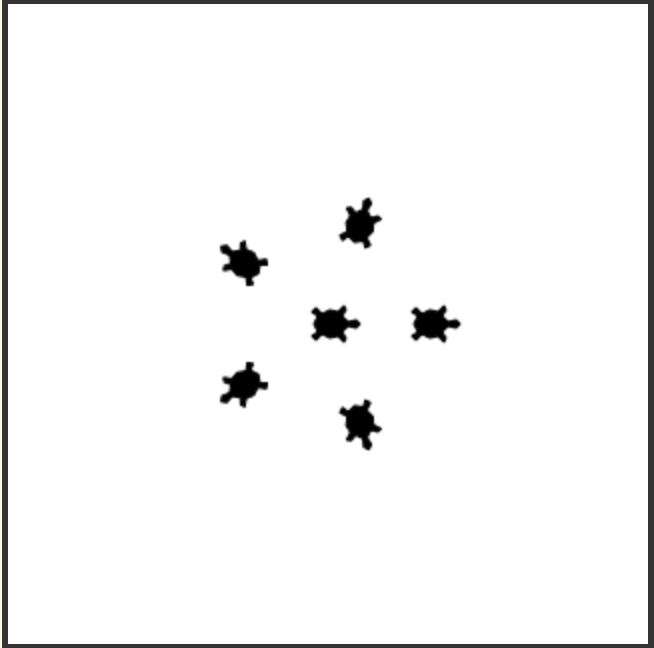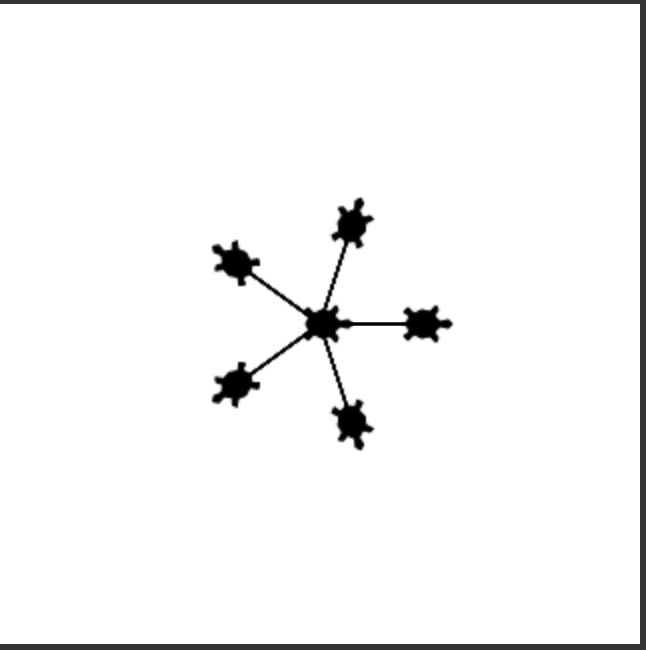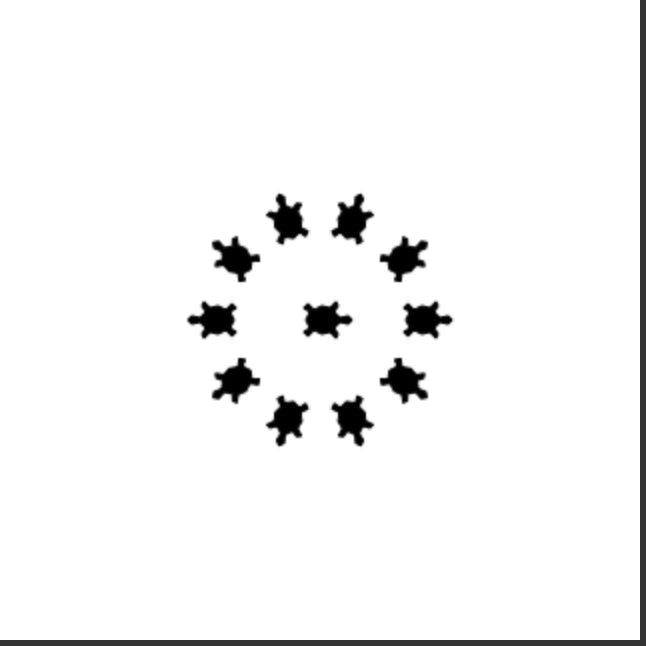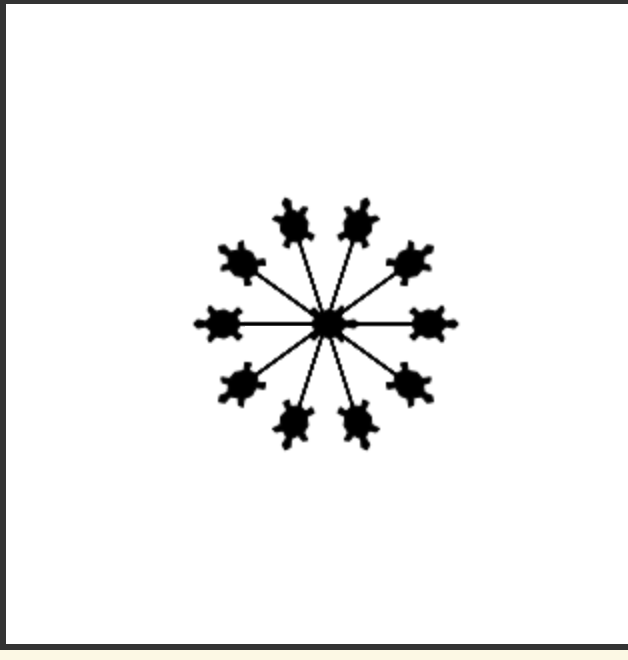# Exam Questions for Chapters 9 to 11¶

The following questions test what you have learned in chapters 9 to 11. Click the “Start” button when you are ready to begin the exam. Click the “Pause” button to pause the exam (you will not be able to see the questions when the exam is paused). It will show how much time you have used, but you have unlimited time. Click on the “Finish Exam” button at the end when you are done. The number correct, number wrong, and number skipped will be displayed at the bottom of the page. Feedback for each answer will also be shown as well as your answer.

You will not be able to change your answers after you hit the “Finish Exam” button.

11-9-1: Given the following code, what will be printed?

```newString = "!"
phrase = "We're off to see the Wizard!"
for letter in phrase:
newString = letter + newString + letter
print (newString)
```
• !We're off to see the Wizard!
• This would be true if it was newString = newString + letter
• !draziW eht ees ot ffo er'eW!
• This would be true if it was newString = letter + newString
• We're off to see the Wizard!!draziW eht ees ot ffo er'eW
• This would be true if it was newString = letter + newString in the loop and you printed phrase + newString
• !draziW eht ees ot ffo er'eW!We're off to see the Wizard!
• This code adds each letter to the front and back of the string which will print the reverse of the string ! and the string.

11-9-2: If we would like to draw a decagon (10 sided figure), what should the values of X and Y be in the code below?

```from turtle import *       # use the turtle library
space = Screen()           # create a turtle space
zoe = Turtle()             # create a turtle named zoe
for sides in range(X):     # repeat the indented lines X times
zoe.forward(50)        # move forward by 50 units
zoe.right(Y)           # And turn each one by Y
```
• X = 5 and Y = 72
• This would be true if you were trying to draw a 5 sided figure.
• X = 36 and Y = 10
• This would be true if you were trying to draw a 36 sided figure.
• X = 10 and Y = 36
• The value of X is 10 to make a 10 sided figure and the amount to turn is 360 / 10 = 36.
• X = 12 and Y = 30
• This would be true if you were trying to draw a 12 sided figure.

11-9-3: Which of the following statements is false?

• Pictures are made up of little pixels, laid out on an (x,y) grid.
• Pictures are grids of pixels.
• Each pixel is stored as a number between 0 and 255.
• A pixel has a color and the color has a red, green, and blue part.
• Each color has a red part, a green part, and a blue part.
• One way to describe a color is a combination of red, green, and blue.
• Each color part is actually a number between 0 and 255.
• On a computer the value for red, green, and blue is between 0 and 255.

11-9-4: What does the following code do?

```from image import *
img = Image("beach.jpg")
pixels = img.getPixels()
for p in pixels:
g = p.getGreen()
b = p.getBlue()
p.setGreen(b / 2)
p.setBlue(g / 2)
img.updatePixel(p)
win = ImageWin(img.getWidth(), img.getHeight())
img.draw(win)
```
• Double the blue and green values in the picture
• This would be true if it was p.setGreen(g * 2) and p.setBlue(b * 2)
• Halve the blue and green values in the picture
• This would be true if it was p.setGreen(g / 2) and p.setBlue(b / 2)
• Set the green values to half the original blue and the blue to half the original green
• This sets the green values to half the original blue values and the blue values to half the original green values.
• Set the green to double half the original blue and the blue to double the original green
• This would be true if it was p.setGreen(b * 2) and p.setBlue(g * 2)

11-9-5: What would the following draw?

```from turtle import *
space = Screen()
zoe = Turtle()
zoe.shape("turtle")
zoe.penup()
for size in range(5):
zoe.forward(50)
zoe.stamp()
zoe.forward(-50)
zoe.right(72)
```
•• This stamps 5 turtles on a circle with a radius of 25.
•• This would be true if the line zoe.penup() was removed.
•• This would be true if it was range(10) and right(36)
•• This would be true if it was range(10) and right(36) and if the line zoe.penup() was removed.